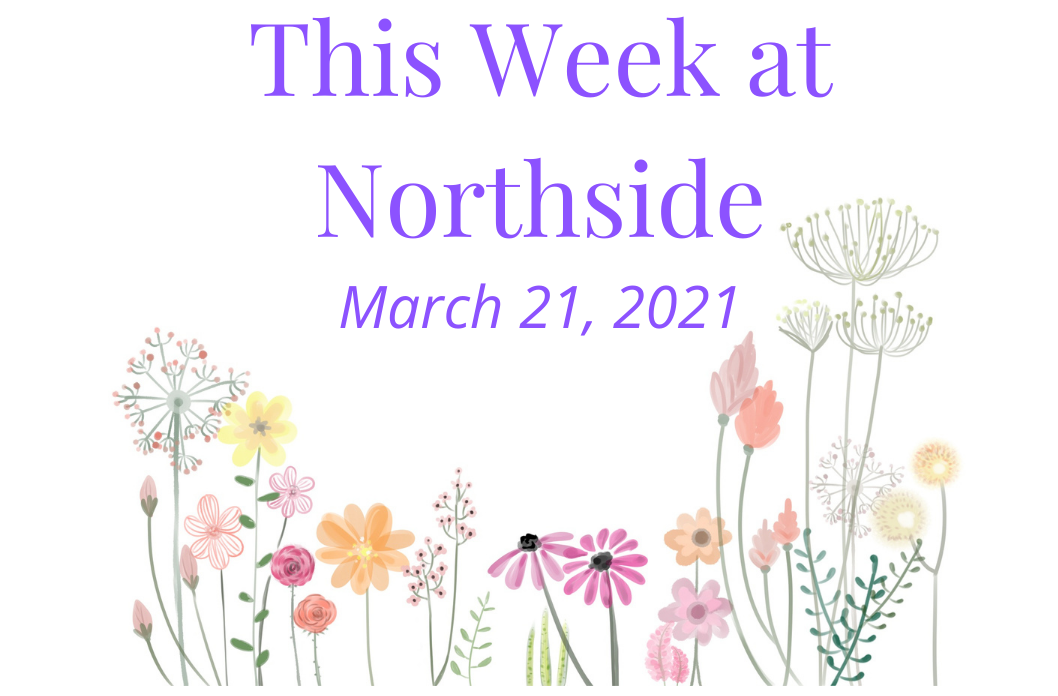/* styles */

# Upcoming Important Dates

Tuesday, March 23 - Friends of Northside Meeting
Thursday, March 25 - Virtual Presentation: Financial Aid Appeals and Professional Judgments
Miércoles 31 de Marzo - Hora de Conversación en Español
Monday, March 29-Friday, April 2 - CPS Spring Break
Friday, April 9 - First Friday

This week's scheduled badminton, soccer, baseball, and volleyball games this week are listed in the Sports article.

 table div table+table+table+table+table div table{width:100%;padding:0}table div table+table+table+table+table div table img{width:96.23%;padding:0;float:none}table div table+table+table+table+table div table td{width:100%;padding:0 1.88% 18px}/* styles */## News from the Counseling Office

▪ NCP Financial Aid Appeals and Professional Judgments Presentation; One-on-One Meetings for Seniors
▪ Follow-Up to Financial Aid Presentation
▪ Update: Summer Enrichment/Pre-College Programs
▪ Chicago Teachers Union Scholarship
▪ Golden Apple Scholars of Illinois
 ▪ NCP Financial Aid Appeals and Professional Judgments Presentation; One-on-One Meetings for Seniors
 ▪ Follow-Up to Financial Aid Presentation
 ▪ Update: Summer Enrichment/Pre-College Programs
 ▪ Chicago Teachers Union Scholarship
 ▪ Golden Apple Scholars of Illinois

## Sports, Yearbook & General School News

▪ LSC Update
▪ Pandemic Electronic Benefit Program
▪ Future Problem Solvers Coaching Opportunity
▪ Annual NCP Fundraiser - Save the Date
▪ Badminton, Soccer, Baseball, and Volleyball Games This Week
▪ Upcoming Registration for Spring Sports: Deadlines for Girls Water Polo, Softball, Baseball, Girls Soccer, Boys & Girls Track & Field, Boys & Girls Lacrosse, Boys Tennis, Boys Volleyball
▪ Available Now: Custom NCP Brick With Your Personalized Message for Your Student
▪ Yearbook Ads for Seniors Available
▪ Order Your 2020-2021 NCP Yearbook
▪ NCP Poms Wins City Championship
▪ Ways for Parents to Stay in Touch/Volunteer/Support NCP/Social Media Links
 ▪ LSC Update
 ▪ Pandemic Electronic Benefit Program
 ▪ Future Problem Solvers Coaching Opportunity
 ▪ Annual NCP Fundraiser - Save the Date
 ▪ Badminton, Soccer, Baseball, and Volleyball Games This Week
 ▪ Upcoming Registration for Spring Sports: Deadlines for Girls Water Polo, Softball, Baseball, Girls Soccer, Boys & Girls Track & Field, Boys & Girls Lacrosse, Boys Tennis, Boys Volleyball
 ▪ Available Now: Custom NCP Brick With Your Personalized Message for Your Student
 ▪ Yearbook Ads for Seniors Available
 ▪ Order Your 2020-2021 NCP Yearbook
 ▪ NCP Poms Wins City Championship
 ▪ Ways for Parents to Stay in Touch/Volunteer/Support NCP/Social Media Links
 table div table+table+table+table+table+table+table div table{width:100%;padding:0}table div table+table+table+table+table+table+table div table img{width:96.23%;padding:0;float:none}table div table+table+table+table+table+table+table div table td{width:100%;padding:0 1.88% 18px}/* styles */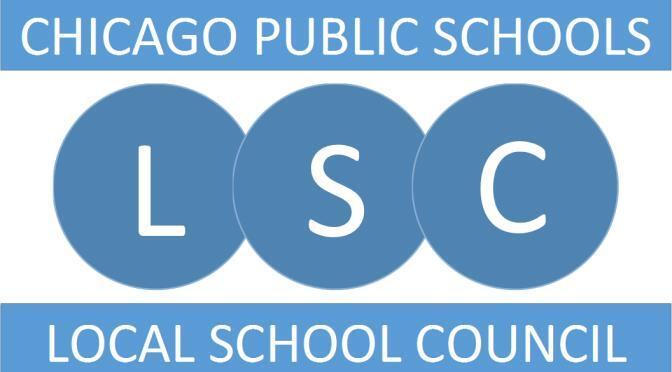# LSC Update

Dear NCP Community,

We want to thank everyone who participated in last week’s forums and/or surveys. We received great feedback that informed the position posting and will assist us as we move into the interview phase of the process.

We especially want to thank the students; 587 responded, 32% of them freshman.

HERE is the principal description that was posted on Wednesday. Feel free to share it with qualified candidates. The application deadline is Wednesday, April 7.

In the meantime, if you have any questions or comments, please email the LSC at northsidecollegepreplsc@gmail.com.

In addition, we have two new LSC members that we appointed at Tuesday’s meeting. Amanda Crowley is our new community representative. She is a teacher-librarian (see her candidate statement.)

In addition, Amy Hennessy is our new parent representative. She is the parent of a freshman and works for the U.S. Department of Health and Human Services (see her candidate statement.)

Our next regularly scheduled meeting is Tuesday, April 20 at 6:30 pm. We hope to see you there.

Sincerely,
~ NCP LSC - David Cihla, Amanda Crowley, Gregory DiFrancesco, Brad Hack, Amy Hennessy, Luna Johnston, John Lynch, Shari Massey, Marion McCreedy, Sandra Morales-Mirque, Martha Mulligan, Erika Schechter, and Patti Stuber

 table div table+table+table+table+table+table+table+table+table div table{width:100%;padding:0}table div table+table+table+table+table+table+table+table+table div table img{width:96.23%;padding:0;float:none}table div table+table+table+table+table+table+table+table+table div table td{width:100%;padding:0 1.88% 18px}/* styles */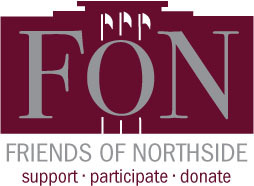# Friends of Northside

Tuesday, March 23

Please join Friends of Northside for their upcoming meeting.

Friends of Northside (FON) is the “one-stop-shop” for NCP families to get involved. FON facilitates communication between parents and the school, organizes parent volunteers, plans and sponsors social/educational events, and raises much-needed funds to bridge the gap due to CPS budget cuts. FON supports the school’s mission to maintain and enhance the learning experience for all students of Northside College Prep High School.

To find out more about Friends of Northside, please visit their website HERE.

 table div table+table+table+table+table+table+table+table+table+table+table div table{width:100%;padding:0}table div table+table+table+table+table+table+table+table+table+table+table div table img{width:96.23%;padding:0;float:none}table div table+table+table+table+table+table+table+table+table+table+table div table td{width:100%;padding:0 1.88% 18px}/* styles */# Hora de Conversación en Español

Miércoles 31 de Marzo
7:00 pm

Se llevará a cabo el miércoles 31 de marzo a las 7:00 pm.

 table div table+table+table+table+table+table+table+table+table+table+table+table+table div table{width:100%;padding:0}table div table+table+table+table+table+table+table+table+table+table+table+table+table div table img{width:96.23%;padding:0;float:none}table div table+table+table+table+table+table+table+table+table+table+table+table+table div table td{width:100%;padding:0 1.88% 18px}/* styles */# Pandemic Electronic Benefit Program

All CPS students, regardless of income or citizenship, are eligible to receive food benefits as part of the US Government’s COVID-19 pandemic response. Funds will be loaded onto cards that will be mailed to each student at the primary address listed for them in ASPEN. To make sure your student receives the benefits check that your primary address is up to date in ASPEN.

Watch the mail for a plain envelope due to arrive this month. Be sure to keep the card because additional benefits will be loaded onto it going forward. For more information, check the March 3 post on SchoolCNXT.

 table div table+table+table+table+table+table+table+table+table+table+table+table+table+table+table div table{width:100%;padding:0}table div table+table+table+table+table+table+table+table+table+table+table+table+table+table+table div table img{width:96.23%;padding:0;float:none}table div table+table+table+table+table+table+table+table+table+table+table+table+table+table+table div table td{width:100%;padding:0 1.88% 18px}/* styles */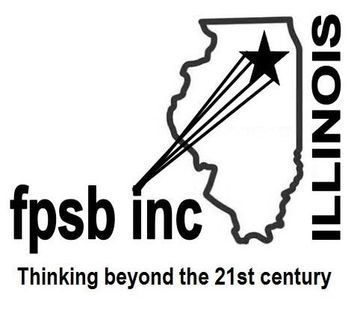# Future Problem Solvers Coaching Opportunity

The Future Problem Solvers Club needs a new coach for next year. FPS teams use the Six-Step Process to create an action plan to solve a problem identified in a scenario of a future situation. The teams of up to four students can compete in regional, state, and international level competitions.

Northside has typically fielded at least one team per grade. Individual competitions are also available. Whether students are interested in the arts and humanities or in STEM subjects, the Six-Step Process allows them to use their strengths in collaboration with other students as they identify challenges and articulate underlying problems, brainstorm possible solutions, generate criteria to evaluate those solutions, and then come up with an action plan to address the problem.

Training is required and provided by FPS Illinois. For more information, contact Janice Cody at jcody6933@gmail.com.

 table div table+table+table+table+table+table+table+table+table+table+table+table+table+table+table+table+table div table{width:100%;padding:0}table div table+table+table+table+table+table+table+table+table+table+table+table+table+table+table+table+table div table img{width:96.23%;padding:0;float:none}table div table+table+table+table+table+table+table+table+table+table+table+table+table+table+table+table+table div table td{width:100%;padding:0 1.88% 18px}/* styles */# Save the date for the annual Northside College Prep Fundraiser

## Friday, May 14, 7:00 pm

Please save the date for the annual Northside College Prep fundraiser. on May 14 at 7 pm. While we can't be together this year to celebrate, we can be "Together but Apart," which is the theme for this year's virtual benefit! We hope you can join us in a fun virtual program showcasing the student talent within our community. Tell your friends, family, and grandparents to join!

And while we have you, please consider helping us with items and experiences for our fundraiser silent auction. We need your help! Start brainstorming something that our families would love, whether it be a local business gift card, time in a vacation home, or another hot item! In addition, if you have a business or company that would make for a good corporate sponsor for the benefit, we would love to have you! Reach out to Linda Weil at Lindaweil-silk@hotmail.com by Sunday, April 25 with ideas for the auction and for corporate sponsorship.

We can't wait to celebrate "together" soon!

 table div table+table+table+table+table+table+table+table+table+table+table+table+table+table+table+table+table+table+table+table div table{width:100%;padding:0}table div table+table+table+table+table+table+table+table+table+table+table+table+table+table+table+table+table+table+table+table div table img{width:96.23%;padding:0;float:none}table div table+table+table+table+table+table+table+table+table+table+table+table+table+table+table+table+table+table+table+table div table td{width:100%;padding:0 1.88% 18px}/* styles */# NCP Financial Aid Appeals and Professional Judgments Presentation; One-on-One Meetings for Seniors

Hello Senior Parents,

As Northside Prep seniors receive their financial aid packages and explore their college options, the counseling department would like to assist your family with navigating the next steps in the financial aid process. The counseling department will offer a Financial Aid Appeals and Professional Judgments virtual presentation on Thursday, March 25 at 6:00 pm. If you are interested in participating in the presentation click here to register.

Please note, the presentation will be facilitated via Google Meet and if you register for the March 25 presentation your student will be required to attend the session with you. Once Ms. Moore receives your meeting request, you will receive a Google Meeting invitation to confirm your registration.

If you are unable to attend the Financial Aid Appeals and Professional Judgments virtual presentation, feel free to schedule a one-on-one meeting with Ms. Moore (click here) via Calendly. During the one-on-one meetings, Ms. Moore is also available to assist students and parents with analyzing their financial aid packages and discussing college options.

## Follow-up: Northside Prep Virtual Financial Aid Presentation

Hello NCP Students and Families,

Thank you for attending the Northside College Prep annual Financial Aid Presentation on Tuesday, March 9th. This year, the counseling department offered the financial aid presentation via Google Meeting and Live Stream. We appreciate your cooperation and patience as we navigate the world of virtual presentations and welcome your feedback about your experience. Click here to complete the virtual financial aid presentation evaluation. Your feedback will be used to enhance the upcoming NCP Financial Aid events and future counseling department events.

As promised, the ISAC and Lawrence University Financial Aid PowerPoint Presentations are attached; click here. I included more details regarding the new CPS graduation requirement and additional links to financial aid resources that may be useful for your family.

If you have any questions or concerns feel free to contact Ms. Moore, NCP College Counselor at mcmoore6@cps.edu.

## Seguimiento: Presentación de ayuda financiera virtual de Northside Prep

Hola, estudiantes y familias de NCP:

Gracias por asistir a la presentación anual de ayuda financiera de Northside College Prep el martes 9 de marzo. Este año, el departamento de asesoramiento ofreció la presentación de ayuda financiera a través de Google Meeting y Live Stream. Agradecemos su cooperación y paciencia mientras navegamos por el mundo de las presentaciones virtuales y agradecemos sus comentarios sobre su experiencia. Haga clic aquí para completar la evaluación de la presentación de ayuda financiera virtual. Sus comentarios se utilizarán para mejorar los próximos eventos de ayuda financiera de NCP y los futuros eventos del departamento de asesoramiento.

Como se prometió, se adjuntan las presentaciones de PowerPoint sobre ayuda financiera de la Universidad de Lawrence y ISAC; haga clic aquí. Incluí más detalles sobre el nuevo requisito de graduación de CPS y enlaces adicionales a recursos de ayuda financiera que pueden ser útiles para su familia.

Si tiene alguna pregunta o inquietud, no dude en comunicarse con la Sra. Moore, consejera universitaria de NCP en mcmoore6@cps.edu.

 table div table+table+table+table+table+table+table+table+table+table+table+table+table+table+table+table+table+table+table+table+table+table+table div table{width:100%;padding:0}table div table+table+table+table+table+table+table+table+table+table+table+table+table+table+table+table+table+table+table+table+table+table+table div table img{width:96.23%;padding:0;float:none}table div table+table+table+table+table+table+table+table+table+table+table+table+table+table+table+table+table+table+table+table+table+table+table div table td{width:100%;padding:0 1.88% 18px}/* styles */# Update: Summer Enrichment/Pre-College Programs

In addition to the virtual information sessions Northside Prep is offering after school daily and during the school day on Wednesdays (click HERE), the counseling department would like to share additional information about information sessions for summer enrichment opportunities.

Are you and your student interested in learning about enrichment opportunities or pre-college programs to engage in during the spring or summer? Would you like to build your resume and enhance your academic, personal, or social skills? If the answer is yes, consider reviewing the following resources to assist your family with identifying the best fit enrichment opportunities for your student:

 table div table+table+table+table+table+table+table+table+table+table+table+table+table+table+table+table+table+table+table+table+table+table+table+table+table+table div table{width:100%;padding:0}table div table+table+table+table+table+table+table+table+table+table+table+table+table+table+table+table+table+table+table+table+table+table+table+table+table+table div table img{width:96.23%;padding:0;float:none}table div table+table+table+table+table+table+table+table+table+table+table+table+table+table+table+table+table+table+table+table+table+table+table+table+table+table div table td{width:100%;padding:0 1.88% 18px}/* styles */# Chicago Teachers Union Scholarship

The Chicago Teachers Union Scholarship Committee is working hard to adapt and maintain our annual tradition of awarding scholarships to children of our members who are graduating and have plans to pursue post-secondary education. If you have a child who will be graduating from high school this year, NOW is the time for them to apply for a CTU/CTUF Scholarship! The deadline is May 10.

Scholarships in the amount of \$1,000 will be awarded to students to help defray the cost of post-secondary education in college or vocational programs. Details are available at www.ctulocal1.org/scholarship.

## Eligibility

Awards are entirely unrestricted as to race, religion, political affiliation, or choice of an accredited college, university, business, trade, or vocational school.

The applicant must be a child of a currently employed, active member in good standing of the Chicago Teachers Union, or a child of a deceased member who was in good standing with the Chicago Teachers Union at the time of death. They must also be a high school senior who will graduate this year before August 31. Special education students are eligible for all scholarships and are encouraged to apply.

## How to Apply

Please make certain that the application is completed in full, and that it is signed by the applicant AND the parent or guardian so that membership in good standing can be verified before presentation to the Scholarship Committee of the Chicago Teachers Union.

## Application Info

Scholarship winners will be notified by mail or email and invited to attend a special virtual reception and award ceremony with their families.

 table div table+table+table+table+table+table+table+table+table+table+table+table+table+table+table+table+table+table+table+table+table+table+table+table+table+table+table+table div table{width:100%;padding:0}table div table+table+table+table+table+table+table+table+table+table+table+table+table+table+table+table+table+table+table+table+table+table+table+table+table+table+table+table div table img{width:96.23%;padding:0;float:none}table div table+table+table+table+table+table+table+table+table+table+table+table+table+table+table+table+table+table+table+table+table+table+table+table+table+table+table+table div table td{width:100%;padding:0 1.88% 18px}/* styles */# Golden Apple Scholars of Illinois

Do you know students in your school or district who would be great teachers? The Golden Apple Scholars teacher preparation and tuition assistance program is accepting applications for the Class of 2021 until April 1!

The Scholars recruitment team will be hosting live information sessions where interested students can learn more about the program, application process, and benefits.

Sessions will be held weekly at 6:00 pm on the following dates:

▪ Tuesday, March 23
▪ Tuesday, March 30

Students can register HERE and we would greatly appreciate your support in sharing this information with students at your school.

▪ Up to \$23,000 in financial assistance for college, inclusive of paid Scholar Institutes
▪ Extensive classroom teaching experience
▪ Job placement assistance
▪ Mentoring from our award-winning teaching faculty

## Who Is Eligible?

▪ Students attending Illinois schools who are high school seniors, freshmen/sophomores in college and community college students
▪ Illinois resident, U.S. citizens, eligible non-citizens, or Illinois Deferred Action for Childhood Arrivals (DACA) students

 table div table+table+table+table+table+table+table+table+table+table+table+table+table+table+table+table+table+table+table+table+table+table+table+table+table+table+table+table+table+table div table{width:100%;padding:0}table div table+table+table+table+table+table+table+table+table+table+table+table+table+table+table+table+table+table+table+table+table+table+table+table+table+table+table+table+table+table div table img{width:96.23%;padding:0;float:none}table div table+table+table+table+table+table+table+table+table+table+table+table+table+table+table+table+table+table+table+table+table+table+table+table+table+table+table+table+table+table div table td{width:100%;padding:0 1.88% 18px}/* styles */# Badminton, Soccer, Baseball, and Volleyball Games This Week

▪ Monday, March 22 - Girls Badminton City Playoffs vs. Roosevelt (away) at 5:00 pm
▪ Wednesday, March 24 - Girls Badminton City Playoffs vs. Roosevelt (away) at 5:00 pm

Boys Soccer
▪ Monday, March 22 vs. Von Steuben @ Lane Stadium (JV at 4:00 pm; V at 5:30 pm)
▪ Saturday, March 27 vs. Westinghouse @ NCP (V at 10:00 am; JV at 11:30 am)

Boys Baseball
▪ Thursday, March 25 at 7:00 pm - player/parent pre-season remote meeting via Google Meet: https://meet.google.com/qze-razj-rop?authuser=0

Girls Volleyball
▪ Tuesday, March 23 vs. Lincoln Park @ Northside (Varsity & Frosh/Soph at 5:00 pm)
▪ Thursday, March 25 vs. Rickover @ Rickover (Varsity & Frosh/Soph at 5:00 pm)

## Videographers Needed

The athletic department is looking for volunteers to help live stream our volleyball matches and possibly other athletic events. Anyone interested in volunteering should contact athletic director Henry Henderson at hhenderson@cps.edu.

 table div table+table+table+table+table+table+table+table+table+table+table+table+table+table+table+table+table+table+table+table+table+table+table+table+table+table+table+table+table+table+table+table div table{width:100%;padding:0}table div table+table+table+table+table+table+table+table+table+table+table+table+table+table+table+table+table+table+table+table+table+table+table+table+table+table+table+table+table+table+table+table div table img{width:96.23%;padding:0;float:none}table div table+table+table+table+table+table+table+table+table+table+table+table+table+table+table+table+table+table+table+table+table+table+table+table+table+table+table+table+table+table+table+table div table td{width:100%;padding:0 1.88% 18px}/* styles */# Upcoming Registration for Spring Sports

## Deadlines for Softball, Baseball, Girls Soccer, Boys & Girls Track & Field, Boys & Girls Lacrosse, Boys Tennis, Boys Volleyball

Registration for softball, baseball, girls soccer, boys & girls track and field, boys & girls lacrosse, boys tennis, and boys volleyball will end on March 22.

Parents can register their students on the NCP athletic website by clicking on the activity registration tab at the top of the page. All students must register and must have a current physical on file in order to try out for a sport at NCP.

The athletic department will publish tryout and practice information as it becomes available. Be sure to look for a message via SchoolCNXT and check the NCP athletic website HERE for any updates.

 table div table+table+table+table+table+table+table+table+table+table+table+table+table+table+table+table+table+table+table+table+table+table+table+table+table+table+table+table+table+table+table+table+table+table div table{width:100%;padding:0}table div table+table+table+table+table+table+table+table+table+table+table+table+table+table+table+table+table+table+table+table+table+table+table+table+table+table+table+table+table+table+table+table+table+table div table img{width:96.23%;padding:0;float:none}table div table+table+table+table+table+table+table+table+table+table+table+table+table+table+table+table+table+table+table+table+table+table+table+table+table+table+table+table+table+table+table+table+table+table div table td{width:100%;padding:0 1.88% 18px}/* styles */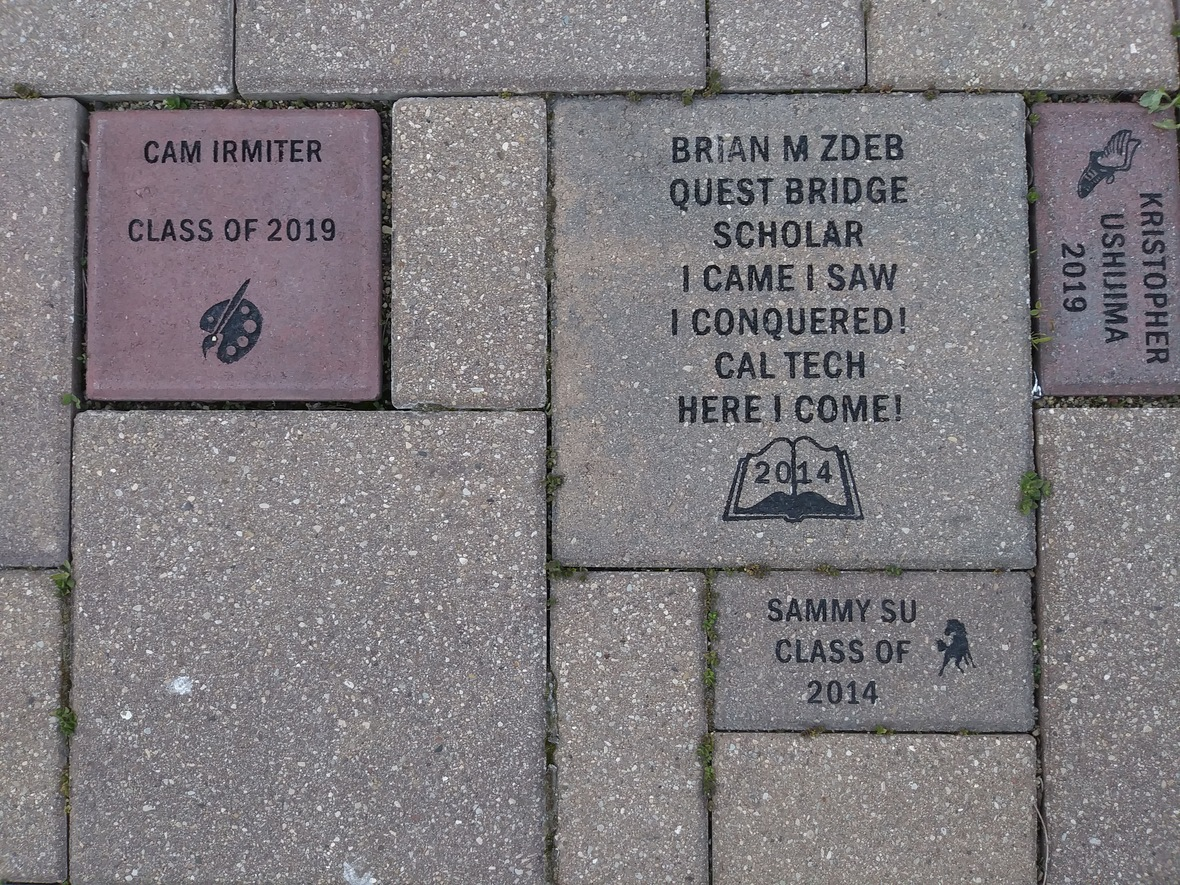Consider buying a custom NCP Brick for your child this year. Your brick, engraved with a personal message, will become a permanent part of the Northside College Prep Celestial Garden Walkway.

Your brick may be purchased in three sizes:

▪ 4" x 8" = \$50
▪ 8" x 8" = \$100
▪ 12" x 12" = \$150

Friends of Northside will provide a gift certificate acknowledging the purchase. Janet Jasmer will assist with the process and can answer any questions at jjasmer@atproperties.com.

 table div table+table+table+table+table+table+table+table+table+table+table+table+table+table+table+table+table+table+table+table+table+table+table+table+table+table+table+table+table+table+table+table+table+table+table+table+table div table{width:100%;padding:0}table div table+table+table+table+table+table+table+table+table+table+table+table+table+table+table+table+table+table+table+table+table+table+table+table+table+table+table+table+table+table+table+table+table+table+table+table+table div table img{width:96.23%;padding:0;float:none}table div table+table+table+table+table+table+table+table+table+table+table+table+table+table+table+table+table+table+table+table+table+table+table+table+table+table+table+table+table+table+table+table+table+table+table+table+table div table td{width:100%;padding:0 1.88% 18px}/* styles */# Any Questions About NCP Communications Sent Via CNXT?

If you are having problems with SchoolCNXT, please contact the main office from 7:30 am - 3:15 pm at 773-534-3954, or you can email the NCP school clerk, Mary Grasz, at mtgrasz@cpu.edu.

NCP uses SchoolCNXT to communicate important information to parents and the school community. All families should have received a text message and/or email communication about signing up. If you did not receive that communication or need access to your student's account, you can gain access by using this tool on Northside's website.

 table div table+table+table+table+table+table+table+table+table+table+table+table+table+table+table+table+table+table+table+table+table+table+table+table+table+table+table+table+table+table+table+table+table+table+table+table+table+table+table+table div table{width:100%;padding:0}table div table+table+table+table+table+table+table+table+table+table+table+table+table+table+table+table+table+table+table+table+table+table+table+table+table+table+table+table+table+table+table+table+table+table+table+table+table+table+table+table div table img{width:96.23%;padding:0;float:none}table div table+table+table+table+table+table+table+table+table+table+table+table+table+table+table+table+table+table+table+table+table+table+table+table+table+table+table+table+table+table+table+table+table+table+table+table+table+table+table+table div table td{width:100%;padding:0 1.88% 18px}/* styles */table.module-40{width:61.89%;padding:0}table div table+table+table+table+table+table+table+table+table+table+table+table+table+table+table+table+table+table+table+table+table+table+table+table+table+table+table+table+table+table+table+table+table+table+table+table+table+table+table+table+table div table{width:61.89%;float:none;margin-left:auto;margin-right:auto;padding:0}table div table+table+table+table+table+table+table+table+table+table+table+table+table+table+table+table+table+table+table+table+table+table+table+table+table+table+table+table+table+table+table+table+table+table+table+table+table+table+table+table+table div table a{border:0 none;text-decoration:none}table div table+table+table+table+table+table+table+table+table+table+table+table+table+table+table+table+table+table+table+table+table+table+table+table+table+table+table+table+table+table+table+table+table+table+table+table+table+table+table+table+table div table img{width:100%!important;border:0 none;text-decoration:none}table div table+table+table+table+table+table+table+table+table+table+table+table+table+table+table+table+table+table+table+table+table+table+table+table+table+table+table+table+table+table+table+table+table+table+table+table+table+table+table+table+table div table td{width:100%;padding:0}/* styles */

# 2020-2021 Yearbook Ads for Seniors Now Available

Seniors now have the opportunity to place ads in the 2020-2021 yearbook by visiting the yearbook website. After clicking on "continue", click on "start your ad" to begin.

 table.module-42{width:68.87%;padding:0}table div table+table+table+table+table+table+table+table+table+table+table+table+table+table+table+table+table+table+table+table+table+table+table+table+table+table+table+table+table+table+table+table+table+table+table+table+table+table+table+table+table+table+table div table{width:68.87%;float:none;margin-left:auto;margin-right:auto;padding:0}table div table+table+table+table+table+table+table+table+table+table+table+table+table+table+table+table+table+table+table+table+table+table+table+table+table+table+table+table+table+table+table+table+table+table+table+table+table+table+table+table+table+table+table div table a{border:0 none;text-decoration:none}table div table+table+table+table+table+table+table+table+table+table+table+table+table+table+table+table+table+table+table+table+table+table+table+table+table+table+table+table+table+table+table+table+table+table+table+table+table+table+table+table+table+table+table div table img{width:100%!important;border:0 none;text-decoration:none}table div table+table+table+table+table+table+table+table+table+table+table+table+table+table+table+table+table+table+table+table+table+table+table+table+table+table+table+table+table+table+table+table+table+table+table+table+table+table+table+table+table+table+table div table td{width:100%;padding:0}/* styles */

# Order a Yearbook Today!

Interested in purchasing a copy of the 2020-2021 Stampede, NCP's yearbook? Click HERE to start your order! And if you didn't get a chance to purchase a copy of the 2019-2020 yearbook, you now have a change to buy one of those, too! Just email Ms. McCreedy at mkmccreedy@cps.edu after you've completed your 2020-2021 order.

Copies are now \$55.

 table div table+table+table+table+table+table+table+table+table+table+table+table+table+table+table+table+table+table+table+table+table+table+table+table+table+table+table+table+table+table+table+table+table+table+table+table+table+table+table+table+table+table+table+table+table+table div table{width:100%;padding:0}table div table+table+table+table+table+table+table+table+table+table+table+table+table+table+table+table+table+table+table+table+table+table+table+table+table+table+table+table+table+table+table+table+table+table+table+table+table+table+table+table+table+table+table+table+table+table div table img{width:96.23%;padding:0;float:none}table div table+table+table+table+table+table+table+table+table+table+table+table+table+table+table+table+table+table+table+table+table+table+table+table+table+table+table+table+table+table+table+table+table+table+table+table+table+table+table+table+table+table+table+table+table+table div table td{width:100%;padding:0 1.88% 18px}/* styles */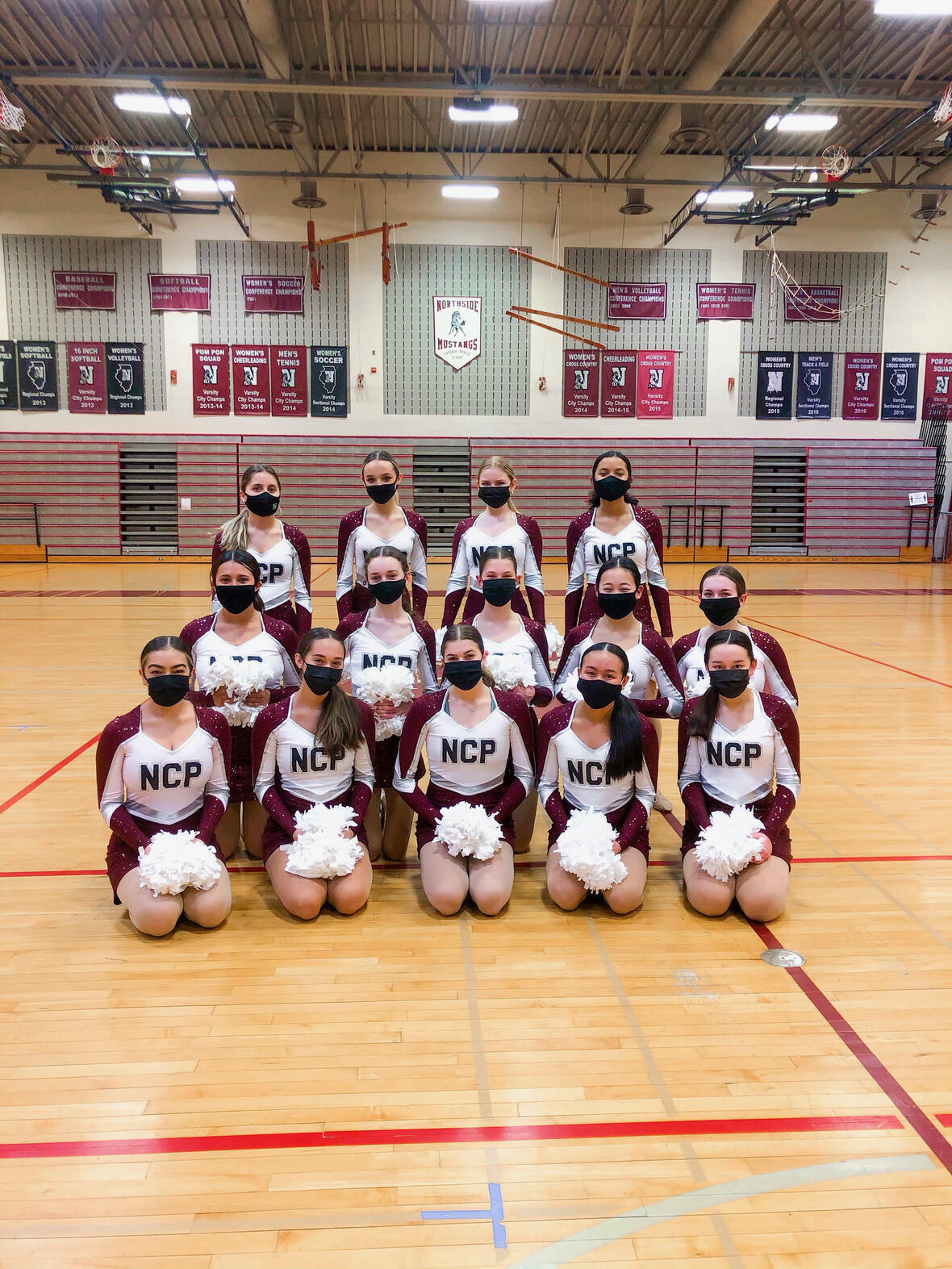# NCP Poms Wins City Championship!

For the second year in a row, NCP's Poms team has won the 2021 City Championship Dance Division 1A champions! Congrats to all the young women who worked so hard to achieve this.

 table div table+table+table+table+table+table+table+table+table+table+table+table+table+table+table+table+table+table+table+table+table+table+table+table+table+table+table+table+table+table+table+table+table+table+table+table+table+table+table+table+table+table+table+table+table+table+table+table+table+table div table{width:100%;padding:0}table div table+table+table+table+table+table+table+table+table+table+table+table+table+table+table+table+table+table+table+table+table+table+table+table+table+table+table+table+table+table+table+table+table+table+table+table+table+table+table+table+table+table+table+table+table+table+table+table+table+table div table img{width:96.23%;padding:0;float:none}table div table+table+table+table+table+table+table+table+table+table+table+table+table+table+table+table+table+table+table+table+table+table+table+table+table+table+table+table+table+table+table+table+table+table+table+table+table+table+table+table+table+table+table+table+table+table+table+table+table+table div table td{width:100%;padding:0 1.88% 18px}/* styles */# Do you have news or team results to share with the Northside parent community?

 table div table+table+table+table+table+table+table+table+table+table+table+table+table+table+table+table+table+table+table+table+table+table+table+table+table+table+table+table+table+table+table+table+table+table+table+table+table+table+table+table+table+table+table+table+table+table+table+table+table+table+table+table div table{width:100%;padding:0}table div table+table+table+table+table+table+table+table+table+table+table+table+table+table+table+table+table+table+table+table+table+table+table+table+table+table+table+table+table+table+table+table+table+table+table+table+table+table+table+table+table+table+table+table+table+table+table+table+table+table+table+table div table img{width:96.23%;padding:0;float:none}table div table+table+table+table+table+table+table+table+table+table+table+table+table+table+table+table+table+table+table+table+table+table+table+table+table+table+table+table+table+table+table+table+table+table+table+table+table+table+table+table+table+table+table+table+table+table+table+table+table+table+table+table div table td{width:100%;padding:0 1.88% 18px}/* styles */# Support Northside - Shop AmazonSmile

AmazonSmile is a simple and automatic way to support Northside every time you shop.

Please note that our AmazonSmile name is Friends of "North" "Side" (North Side - two words at AmazonSmile).

 table div table+table+table+table+table+table+table+table+table+table+table+table+table+table+table+table+table+table+table+table+table+table+table+table+table+table+table+table+table+table+table+table+table+table+table+table+table+table+table+table+table+table+table+table+table+table+table+table+table+table+table+table+table+table div table{width:100%;padding:0}table div table+table+table+table+table+table+table+table+table+table+table+table+table+table+table+table+table+table+table+table+table+table+table+table+table+table+table+table+table+table+table+table+table+table+table+table+table+table+table+table+table+table+table+table+table+table+table+table+table+table+table+table+table+table div table img{width:96.23%;padding:0;float:none}table div table+table+table+table+table+table+table+table+table+table+table+table+table+table+table+table+table+table+table+table+table+table+table+table+table+table+table+table+table+table+table+table+table+table+table+table+table+table+table+table+table+table+table+table+table+table+table+table+table+table+table+table+table+table div table td{width:100%;padding:0 1.88% 18px}/* styles */# Follow Us on Social Media

 table div table+table+table+table+table+table+table+table+table+table+table+table+table+table+table+table+table+table+table+table+table+table+table+table+table+table+table+table+table+table+table+table+table+table+table+table+table+table+table+table+table+table+table+table+table+table+table+table+table+table+table+table+table+table+table+table div table{width:100%;padding:0}table div table+table+table+table+table+table+table+table+table+table+table+table+table+table+table+table+table+table+table+table+table+table+table+table+table+table+table+table+table+table+table+table+table+table+table+table+table+table+table+table+table+table+table+table+table+table+table+table+table+table+table+table+table+table+table+table div table img{width:96.23%;padding:0;float:none}table div table+table+table+table+table+table+table+table+table+table+table+table+table+table+table+table+table+table+table+table+table+table+table+table+table+table+table+table+table+table+table+table+table+table+table+table+table+table+table+table+table+table+table+table+table+table+table+table+table+table+table+table+table+table+table+table div table td{width:100%;padding:0 1.88% 18px}/* styles */table div table+table+table+table+table+table+table+table+table+table+table+table+table+table+table+table+table+table+table+table+table+table+table+table+table+table+table+table+table+table+table+table+table+table+table+table+table+table+table+table+table+table+table+table+table+table+table+table+table+table+table+table+table+table+table+table+table+table div table{width:100%;padding:0}table div table+table+table+table+table+table+table+table+table+table+table+table+table+table+table+table+table+table+table+table+table+table+table+table+table+table+table+table+table+table+table+table+table+table+table+table+table+table+table+table+table+table+table+table+table+table+table+table+table+table+table+table+table+table+table+table+table+table div table img{width:96.23%;padding:0;float:none}table div table+table+table+table+table+table+table+table+table+table+table+table+table+table+table+table+table+table+table+table+table+table+table+table+table+table+table+table+table+table+table+table+table+table+table+table+table+table+table+table+table+table+table+table+table+table+table+table+table+table+table+table+table+table+table+table+table+table div table td{width:100%;padding:0 1.88% 18px}/* styles */table div table+table+table+table+table+table+table+table+table+table+table+table+table+table+table+table+table+table+table+table+table+table+table+table+table+table+table+table+table+table+table+table+table+table+table+table+table+table+table+table+table+table+table+table+table+table+table+table+table+table+table+table+table+table+table+table+table+table+table+table div table{width:100%;padding:0}table div table+table+table+table+table+table+table+table+table+table+table+table+table+table+table+table+table+table+table+table+table+table+table+table+table+table+table+table+table+table+table+table+table+table+table+table+table+table+table+table+table+table+table+table+table+table+table+table+table+table+table+table+table+table+table+table+table+table+table+table div table img{width:96.23%;padding:0;float:none}table div table+table+table+table+table+table+table+table+table+table+table+table+table+table+table+table+table+table+table+table+table+table+table+table+table+table+table+table+table+table+table+table+table+table+table+table+table+table+table+table+table+table+table+table+table+table+table+table+table+table+table+table+table+table+table+table+table+table+table+table div table td{width:100%;padding:0 1.88% 18px}/* styles */